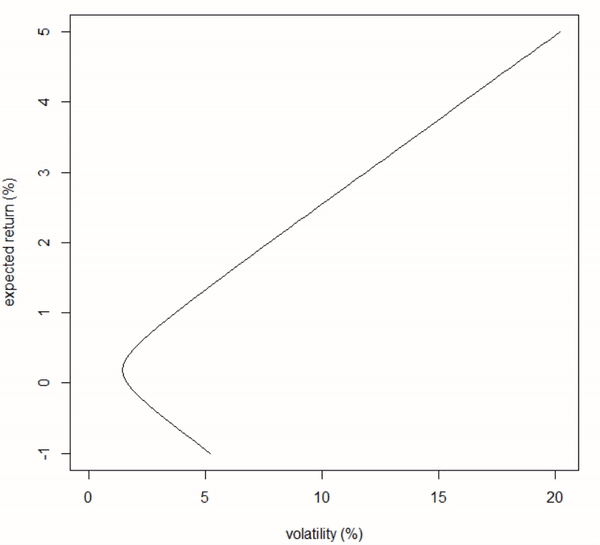# Incremental efficient frontier

In this tutorial, I quickly describe how to compute and update an efficient frontier in adding stocks to an existing porfolio with R.

## Step 1: Efficient frontier

First let’s write a simple code for an efficient frontier computation

### Code

``````
efficient_frontier = function(MRet                         #matrix of returns (MRet)
, rangeMu)                   #range (sequence) of target expected returns (rangeMu)
{

uM <- dim(MRet)                                       #get the row (uM) and column (pM) dimensions of the matrix of returns
pM <- dim(MRet)

expRet <- colMeans(MRet)                                 #compute the portfolio's individual stocks expected returns

Omega = var(MRet)                                        #compute the sample var-covar matrix

unityVec <- rep(1, pM)                                   #define a constraints vector (weights of the portfolio must sum to one)

A <- rbind(expRet, unityVec)                             #define a matrix of constraints (weights sum to one and variance will match a                                                                   #target level of expected returns)

n <- length(rangeMu)                                     #get the length of the target range (sequence)

myVar <- rep(NA, n)                                      #define an empty variance vector

myWeights <- matrix(data = NA, nrow = n, ncol = pM)      #define an empty matrix of weights for each stock at each level of target
#expected returns

for(i in 1:n)                                            #loop over the target expected returns range and compute variances and weights
{
b <- matrix(data = c(rangeMu[i], 1), nrow = 2)
myVar[i] <- t(b) %*% solve(A %*% solve(Omega) %*% t(A)) %*% b
myWeights[i,] <- solve(Omega) %*% t(A) %*% solve(A %*% solve(Omega) %*% t(A)) %*% b
}

mySd <- myVar^0.5                                        #compute the standard deviation vector

return(mySd)                                             #return the standard deviation vector
}``````

## Step 2: Incremental efficient frontier

### Code

``````
efficient_frontier_increment = function(MRet)                      #define a function that takes as argument a matrix of returns (MRet)
{
pM <- dim(MRet)                                               #get the column (pM) dimension of the matrix of returns

rangeMu <- seq(-0.01, 0.05, 0.001)                               #hard code the vector of expected returns (can be passed as argument)

mySd <- efficient_frontier(MRet[, 1:9], rangeMu)                 #compute the sd vector of the portfolio composed of the first nine stocks

dev.new()                                                        #get a new plot

plot(mySd * 100                                                  #plot the efficient frontier of the portfolio composed of the first 9 stocks
, rangeMu * 100
, xlab = "volatility (%)"
, ylab = "expected return (%)"
, t = 'l'
, xlim = c(0, max(mySd * 100))
, ylim = c(-1, 5))

Sys.sleep(2)                                                     #enjoy the chart for two seconds

for(j in 10:pM)                                                  #loop over all stocks and increment the portfolio with the next stock
{
mySd <- efficient_frontier(MRet[, 1:j], rangeMu)               #recompute the efficient frontier

lines(mySd * 100, rangeMu * 100, col = 'red')                  #add a line of the more efficient frontier on the chart

Sys.sleep(0.1)                                                 #define how long you want to wait before the next one
}
}``````

## Result in animationFig 1. Animation of an efficient frontier with stock increment

To run the code with real historical figures, I propose a *.csv file of NYSE returns